# Particle Balance

Let us now examine particle balance in a thermonuclear plasma trapped in a magnetic confinement device. The number densities of the deuterons, tritons, and helium ash particles in the plasma evolve in time according to the following idealized equations: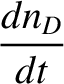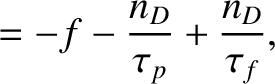(1.27)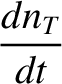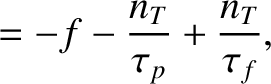(1.28)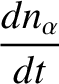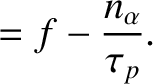(1.29)

Here,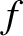is the rate of nuclear fusion reactions per unit volume. [See Equation (1.6).] Moreover,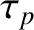is the particle confinement time, which is defined as the average time that a particle is confined within the plasma. The deuterons, tritons, and helium ash particles are assumed to have the same particle confinement times, for the sake of simplicity. Finally,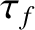is the fueling time, which is the average time on which fusion reagents are replenished in the plasma (e.g., via the injection of frozen deuterium/tritium pellets) . We are assuming that deuterons and tritons are replenished at the same rate. Of course, there is no helium ash particle fueling (other than that provided by nuclear fusion reactions).

Suppose that the plasma has attained a steady state (i.e.,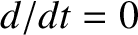). Let us assumed that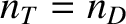(i.e., the deuterium-tritium mix is optimal for nuclear fusion). The burn fraction,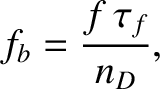(1.30)

is defined as the fraction of fuel ions injected into the plasma that undergo fusion reactions, rather than escaping. Equations (1.27) and (1.29) imply that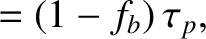(1.31)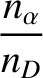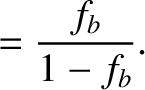(1.32)

Quasi-neutrality  demands that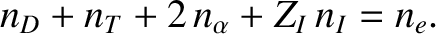(1.33)

Here, we are supposing that the plasma contains a fixed fraction of impurity ions of number density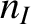and mean charge number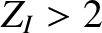. Usually, such ions originate from the interaction between the plasma and the surrounding solid surfaces. Typical impurity ion species are beryllium, boron, carbon, or tungsten. The effective charge number of the plasma ions is defined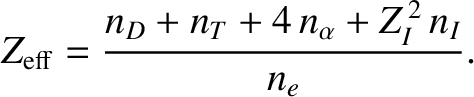(1.34)

The pervious six equations can be combined with Equation (1.6) to give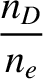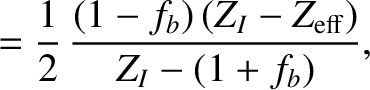(1.35)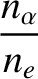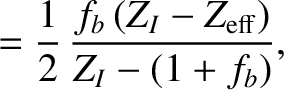(1.36)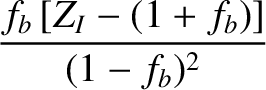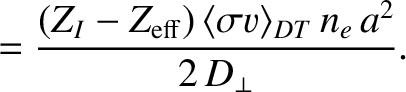(1.37)

Here, we have written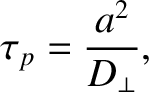(1.38)

where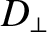is the mean rate at which particles diffuse through the plasma. In practice, particles diffuse out of a magnetic confinement device under the action of small-scale turbulence driven by density and temperature gradients within the plasma [16,64]. Moreover, the typical particle diffusion rate is about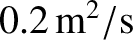.

Table 1.3: More characteristic properties of a thermonuclear plasma trapped in a low-field and a high-field magnetic confinement device. Here,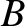is the magnetic field-strength,the particle confinement time,the fueling time,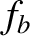the burn fraction,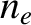the electron number density,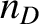the deuteron number density,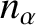the alpha particle number density,the impurity ion number density, and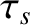the alpha particle slowing-down time.
 Low-Field High-Field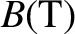5.0 12.0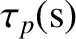31 6.1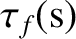30 5.7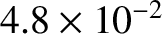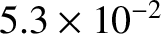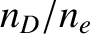0.433 0.431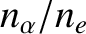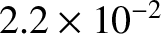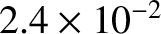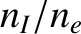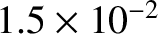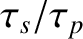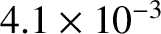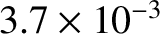Assuming that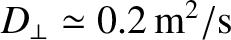,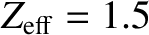[14,55], and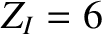(which corresponds to fully-stripped carbon impurity ions), making use of the data given in Table 1.2, and employing Equations (1.31) and (1.34)–(1.38), we can derive the particle balance data given in Table 1.3. [Incidentally, Equation (1.37) is most easily solved forvia iteration.] Note that, for both low-field and high-field confinement devices, the burn fraction is only about 5%. In other words, only 5% of the fuel ions injected into the plasma undergo nuclear fusion reactions; the remainder escape. Unfortunately, this is necessary, otherwise there would be an unacceptable build up of helium ash within the plasma. [See Equation (1.32).] In fact, we estimate the helium ash to make up about 3% percent of the ion content of the plasma. The presence of helium ash and impurity ions in the plasma dilutes the concentration of fuel ions, with respect to that which would be present if the helium ash and impurities were absent, by about 14%. This, in turn, reduces the nuclear fusion reaction rate in the plasma by about 25%. We conclude that the helium ash and impurity ion contents of the plasma must be kept as low as possible. Incidentally heavy impurity ions, such as tungsten, have such a high mean charge number in a thermonuclear plasma (about 60, in the case of tungsten) that their relative concentration must be significantly less than that given in Table 1.3 (about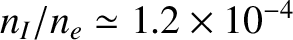, in the case of tungsten) in order to avoid unacceptable fuel ion dilution.

If thermonuclear fusion is to work properly then the time required for alpha particle fusion products to slow down due to collisions with thermal particles, and, thereby, heat the plasma, must be much less than the particle confinement time. (Here, we are making the simplifying assumption that thermonuclear alpha particles have the same confinement time as the thermalized plasma species.) Otherwise, alpha particles would be able to escape from the plasma without giving up all of their fusion energy. The slowing-down time for alpha particles is given approximately by [21,57,58]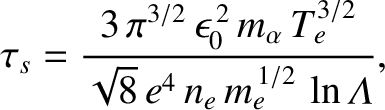(1.39)

where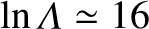is the Coulomb logarithm , and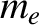the electron mass. As shown in Table 1.3, the ratiois indeed very much less than unity in thermonuclear magnetic confinement devices.